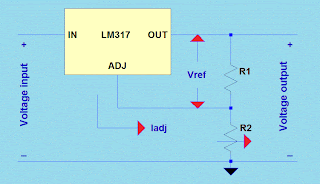### 3LM317 Calculator

This calculator helps you set the output voltage of LM317
regulator IC by simply replacing the value of both R1 and R2.
The value of R1 usually varies from 100 t0 1000 ohms while
R2 is of any value and preferably a trimmer type or potentiometer.

The output voltaged desired can be calculated using this formula.

where Vref = 1.25V and Iadj = 100uA.

The first calculator allows you to set the value of both R1 and R2
to determine the output voltage regulated by LM317.
The next calculator allows you to set your desired output voltage and
value of R1 to determine the value of R2.lm317 regulator

 R1 resistor R2 resistor Output Voltage Voltage out R1 resistor R2 value

1.This comment has been removed by a blog administrator.

2.This comment has been removed by the author.

3.What is the input voltage to this?